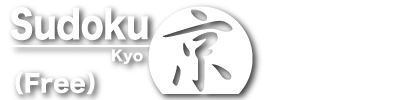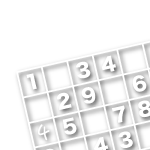Sponsored Links

TOP

# Solving Basics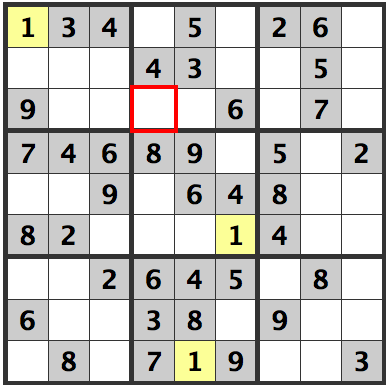Here are the basics. In the picture there is a 1 occupying the first row in the upper left. Because there are already 1's in the second and third columns of the center stack, we can confirm that a 1 goes in the cell indicated in red in the upper section.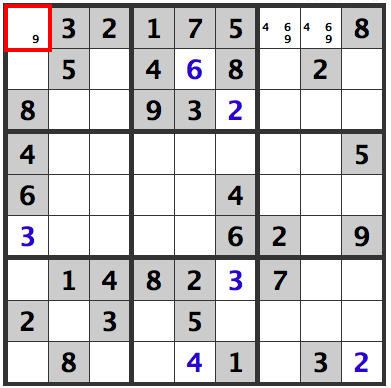In the uppermost row, there are three cells open. 4, 6, and 9 are all possible candidates, but there are already a 4 and a 6 in the leftmost column. The cell indicated in red must be a 9.

Sponsored Links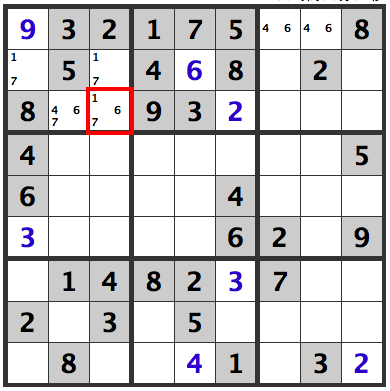1, 4, 6, and 7 are all possibilities for the four open cells in the upper left box, but since 4 and 6 are already present in the middle section of the second row, the two open cells in the second row must be either 1 and 7, or 7 and 1. Either way, since we know that those two cells will use 1 and 7, we know that 1 and 7 cannot appear in the cell indicated in red. Since there is a 4 below that cell, there must be a 6 here, which means that the cell next to it must be a 4.Following the pattern of the 1 and 7 in the upper left box, the candidates for the upper right box are 1, 3, 7, and 9. However, we already know that 1 and 7 are already in the upper left box, so 1 and 7 cannot appear in the cell indicated in red. Since there is a 9 below that cell, this must be a 3, which means that the other cell must be a 9.

Sponsored Links